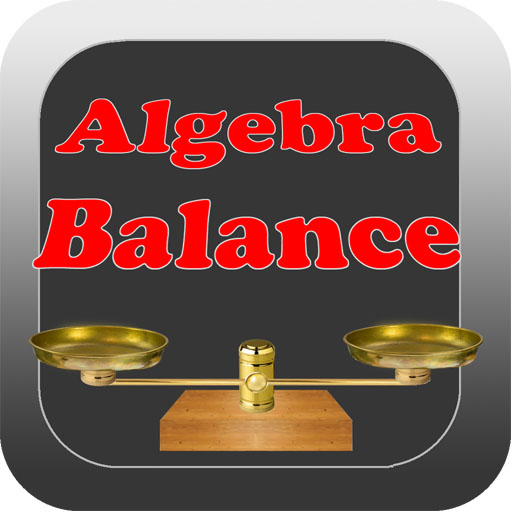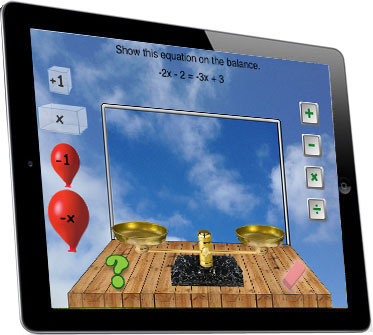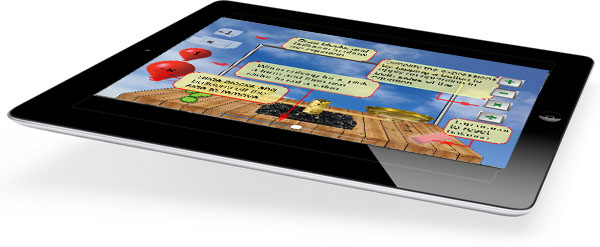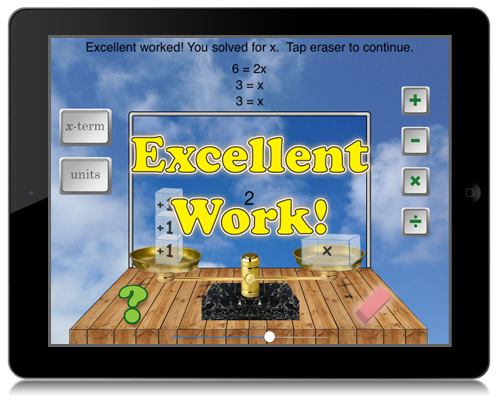Math Power Series Introduce Methods for Solving for x Using an Interactive Visual ToolAlgebra Balance provides teachers and parents with an educational tool for demonstrating and practicing methods for solving for x given an algebraic equation with one variable. Using the Algebra Balance teachers will want to encourage students to build expressions using blocks and balloons. Blocks represent positive values and balloons represent negative values. Once two equal expressions are shown, the student is challenged to analyze the equation and then begin a procedure for simplification in order to find the value of x that will solve the equation. Active learning begins by moving blocks or balloons onto the balance scale. By choosing the appropriate terms the student defines two expressions. Interactively the balance shows the relationship between the two expressions. The goal is for the student to solve for x by manipulating the balance. Terms are added and subtracted from both sides and the results are immediately displayed on the screen.Visualization helps make algebra less abstract. By representing equivalent expressions on the left and right side of the balance students develop an intuitive understanding of algebra.A help screen informs students about how to operate the app.By adding x's and units to both sides of the balance the students visually solves for x. Once x is isolated on one side of the equation a message pops up to inform the student that the equation has been solved.
 \$1.99